# Title 40

## SECTION 53.56

### 53.56 Test for effect of variations in ambient pressure.

§ 53.56 Test for effect of variations in ambient pressure.

(a) Overview. (1) This test procedure is designed to test various sampler performance parameters under variations in ambient (barometric) pressure. Tests shall be conducted in a pressure-controlled environment over two 6-hour time periods during which reference pressure and flow rate measurements shall be made at intervals not to exceed 5 minutes. Specific parameters to be evaluated at operating pressures of 600 and 800 mm Hg are as follows:

(i) Sample flow rate.

(ii) Flow rate regulation.

(iii) Flow rate measurement accuracy.

(iv) Coefficient of variability measurement accuracy.

(v) Ambient pressure measurement accuracy.

(vi) Proper operation of the sampler when exposed to ambient pressure extremes.

(2) The performance parameters tested under this procedure, the corresponding minimum performance specifications, and the applicable test conditions are summarized in table E-1 of this subpart. Each performance parameter tested, as described or determined in the test procedure, must meet or exceed the associated performance specification given. The candidate sampler must meet all specifications for the associated PM 2.5 or PM 10-2.5 method (as applicable) to pass this test procedure.

(b) Technical definition. Sample flow rate means the quantitative volumetric flow rate of the air stream caused by the sampler to enter the sampler inlet and pass through the sample filter, measured in actual volume units at the temperature and pressure of the air as it enters the inlet.

(c) Required test equipment. (1) Hypobaric chamber or other pressure-controlled environment or environments, capable of obtaining and maintaining pressures at 600 mm Hg and 800 mm Hg required for the test with an accuracy of 5 mm Hg. Henceforth, where the test procedures specify a test or environmental chamber, an alternative pressure-controlled environmental area or areas may be substituted, provided the test pressure requirements are met. Means for simulating ambient pressure using a closed-loop sample air system may also be approved for this test; such a proposed method for simulating the test pressure conditions may be described and submitted to EPA at the address given in § 53.4(a) prior to conducting the test for a specific individual determination of acceptability.

(2) Flow rate meter, suitable for measuring and recording the actual volumetric sampler flow rate at the sampler downtube, with a minimum range of 10 to 25 L/min, 2 percent certified, NIST-traceable accuracy. Optional capability for continuous (analog) recording capability or digital recording at intervals not to exceed 5 minutes is recommended. While a flow meter which provides a direct indication of volumetric flow rate is preferred for this test, an alternative certified flow measurement device may be used as long as appropriate volumetric flow rate corrections are made based on measurements of actual ambient temperature and pressure conditions.

(3) Ambient air temperature recorder (if needed for volumetric corrections to flow rate measurements) with a range −30 °C to = 50 °C, certified accurate to within 0.5 °C. If the certified flow meter does not provide direct volumetric flow rate readings, ambient temperature measurements must be made using continuous (analog) recording capability or digital recording at intervals not to exceed 5 minutes.

(4) Barometer, range 600 mm Hg to 800 mm Hg, certified accurate to 2 mm Hg. Ambient air pressure measurements must be made using continuous (analog) recording capability or digital recording at intervals not to exceed 5 minutes.

(5) Flow measurement adaptor (40 CFR part 50, appendix L, figure L-30) or equivalent adaptor to facilitate measurement of sampler flow rate at the sampler downtube.

(6) Means for creating an additional pressure drop of 55 mm Hg in the sampler to simulate a heavily loaded filter, such as an orifice or flow restrictive plate installed in the filter holder or a valve or other flow restrictor temporarily installed in the flow path near the filter.

(7) Teflon sample filter, as specified in section 6 of 40 CFR part 50, appendix L (if required).

(d) Calibration of test measurement instruments. Submit documentation showing evidence of appropriately recent calibration, certification of calibration accuracy, and NIST-traceability (if required) of all measurement instruments used in the tests. The accuracy of flow rate meters shall be verified at the highest and lowest pressures and temperatures used in the tests and shall be checked at zero and at least one flow rate within ±3 percent of 16.7 L/min within 7 days prior to use for this test. Where an instrument's measurements are to be recorded with an analog recording device, the accuracy of the entire instrument-recorder system shall be calibrated or verified.

(e) Test setup. (1) Setup of the sampler shall be performed as required in this paragraph (e) and otherwise as described in the sampler's operation or instruction manual referred to in § 53.4(b)(3). The sampler shall be installed upright and set up in the pressure-controlled chamber in its normal configuration for collecting PM samples. A sample filter and (or) the device for creating an additional 55 mm Hg pressure drop shall be installed for the duration of these tests. The sampler's ambient temperature, ambient pressure, and flow measurement systems shall all be calibrated per the sampler's operating manual within 7 days prior to this test.

(2) The inlet of the candidate sampler shall be removed and the flow measurement adaptor installed on the sampler's downtube. A leak check as described in the sampler's operation or instruction manual shall be conducted and must be properly passed before other tests are carried out.

(3) The inlet of the flow measurement adaptor shall be connected to the outlet of the flow rate meter.

(4) The barometer shall be installed in the test chamber such that it will accurately measure the air pressure to which the candidate sampler is subjected.

(f) Procedure. (1) Set up the sampler as specified in paragraph (e) of this section and otherwise prepare the sampler for normal sample collection operation as directed in the sampler's operation or instruction manual.

(2) The test shall consist of two test runs, one at each of the following conditions of chamber pressure:

(i) 600 mm Hg.

(ii) 800 mm Hg.

(3) For each of the two test runs, set the selected chamber pressure for the test run. Upon achieving each pressure setpoint in the chamber, the candidate sampler shall be pressure-equilibrated for a period of at least 30 minutes prior to the test run. Following the conditioning time, set the sampler to automatically start a 6-hour sample collection period at a convenient time.

(4) During each 6-hour test period:

(i) Measure and record the sample flow rate with the flow rate meter at intervals not to exceed 5 minutes. If ambient temperature and pressure corrections are necessary to calculate volumetric flow rate, ambient temperature and pressure shall be measured at the same frequency as that of the certified flow rate measurements. Note and record the actual start and stop times for the 6-hour flow rate test period.

(ii) Determine and record the ambient (chamber) pressure indicated by the sampler and the corresponding ambient (chamber) pressure measured by the barometer specified in paragraph (c)(4) of this section at intervals not to exceed 5 minutes.

(5) At the end of each test period, terminate the sample period (if not automatically terminated by the sampler) and download all archived instrument data for the test run from the test sampler.

(g) Test results. For each of the two test runs, examine the chamber pressure measurements. Verify that the pressure met the requirements specified in paragraph (f) of this section at all times during the test. If not, the test run is not valid and must be repeated. Determine the test results as follows:

(1) Mean sample flow rate. (i) From the certified measurements (Qref) of the test sampler flow rate, tabulate each flow rate measurement in units of L/min. If ambient temperature and pressure corrections are necessary to calculate volumetric flow rate, each measured flow rate shall be corrected using its corresponding temperature and pressure measurement values. Calculate the mean flow rate for the sample period (Qref,ave) as follows:

Equation 17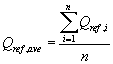where: n equals the number of discrete certified flow measurements over the 6-hour test period.

(ii)(A) Calculate the percent difference between this mean flow rate value and the design value of 16.67 L/min, as follows:

Equation 18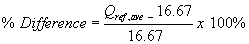(B) To successfully pass this test, the percent difference calculated in Equation 18 of this paragraph (g)(1) must be within ±5 percent for each test run.

(2) Sample flow rate regulation. (i) From the certified measurements of the test sampler flow rate, calculate the sample coefficient of variation of the discrete measurements as follows:

Equation 19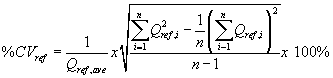(ii) To successfully pass this test, the calculated coefficient of variation for the certified flow rates must not exceed 2 percent.

(3) Flow rate measurement accuracy. (i) Using the mean volumetric flow rate reported by the candidate test sampler at the completion of each 6-hour test (Qind,ave), determine the accuracy of the reported mean flow rate as:

Equation 20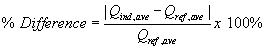(ii) To successfully pass this test, the percent difference calculated in Equation 20 of this paragraph (g)(3) shall not exceed 2 percent for each test run.

(4) Flow rate CV measurement accuracy. (i) Using the flow rate coefficient of variation indicated by the candidate test sampler at the completion of the 6-hour test (%CVind), determine the accuracy of the reported coefficient of variation as:

Equation 21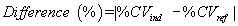(ii) To successfully pass this test, the absolute difference in values calculated in Equation 21 of this paragraph (g)(4) must not exceed 0.3 (CV%) for each test run.

(5) Ambient pressure measurement accuracy. (i) Calculate the absolute difference between the mean ambient air pressure indicated by the test sampler and the ambient (chamber) air pressure measured with the reference barometer as:

Equation 22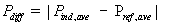where: Pind,ave = mean ambient pressure indicated by the test sampler, mm Hg; and Pref,ave = mean barometric pressure measured by the reference barometer, mm Hg.

(ii) The calculated pressure difference must be less than 10 mm Hg for each test run to pass the test.

(6) Sampler functionality. To pass the sampler functionality test, the following two conditions must both be met for each test run:

(i) The sampler must not shut down during any part of the 6-hour tests; and

(ii) An inspection of the downloaded data from the test sampler verifies that all the data are consistent with normal operation of the sampler.

[62 FR 38799, July 18, 1997; 63 FR 7714, Feb. 17, 1998, as amended at 71 FR 61292, Oct. 17, 2006]